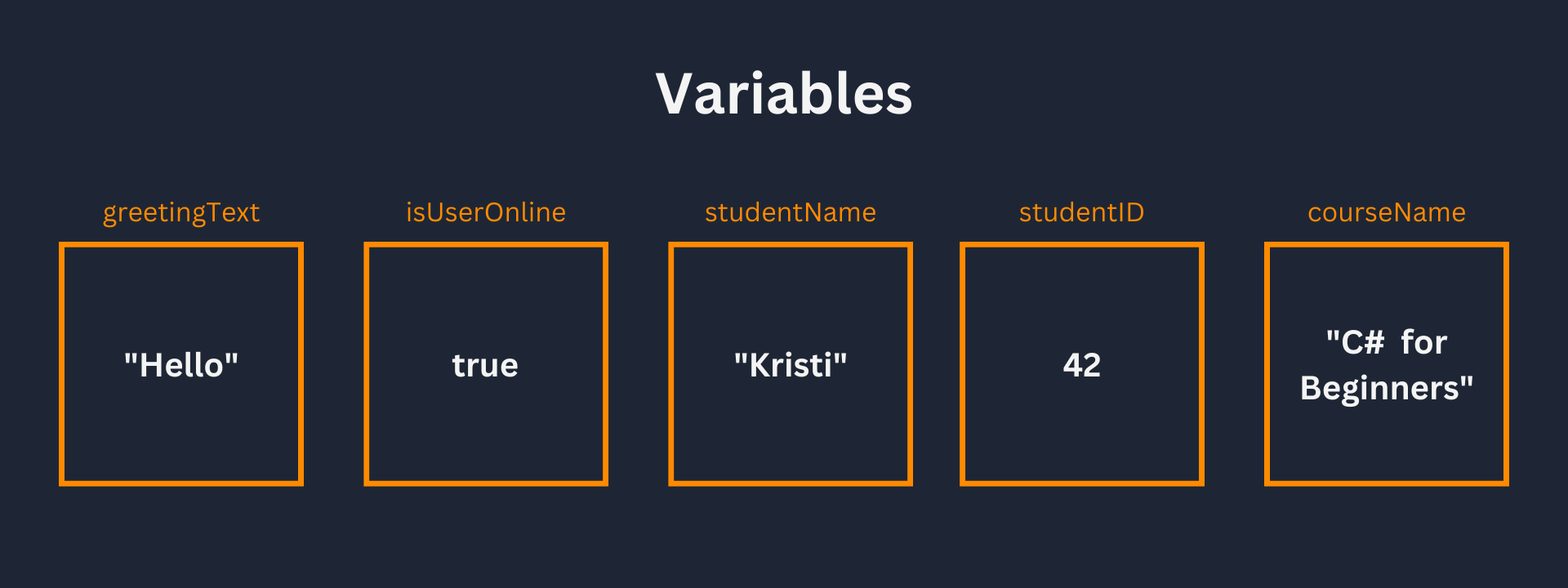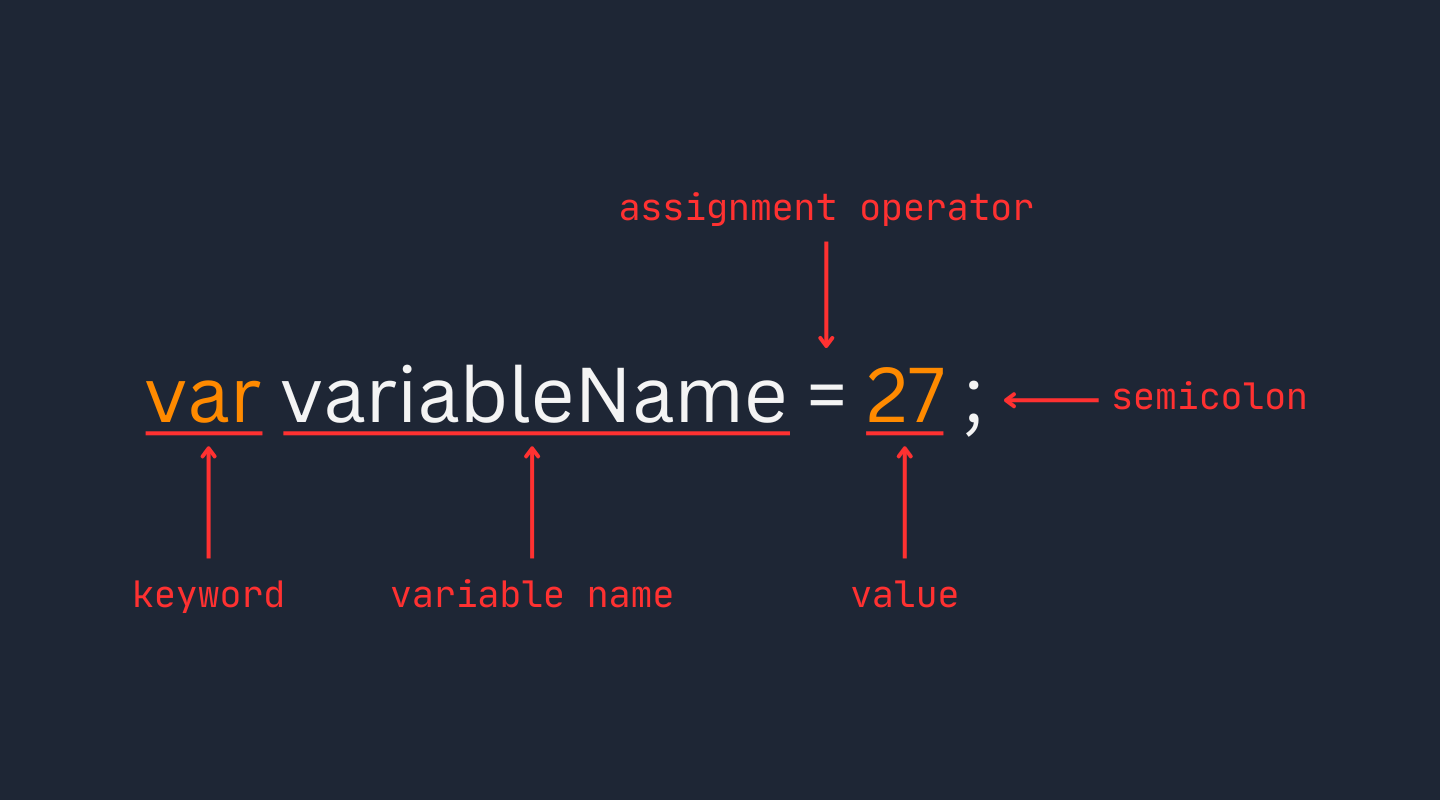Course Content

C# Basics

##Variables

In programming, variables are like labeled containers that hold some value. The data may be in the form of a number, text, boolean (true / false) or some other type.The term "variable" means "changeable" hence the value that a variable holds can be changed any time.

We can create a simple variable in C# using the `var` keyword:

The different components of this syntax are highlighted in the graphic below:The equal sign (`=`) is called the assignment operator. It is used for assigning values to variables.

We will only use numbers for values of variables in this section. In the next section, we will learn how to work with different types of data in appropriate detail.

Note

A statement which includes `=` operator is called an Assignment Statement, for-example: `variableName = value`.

For-example:main.csThis above code creates a new variable called `myVar` which holds the value `10`. It then outputs the value of the variable using the `System.Console.WriteLine` statement.

We can change the value it holds using the assignment operator:main.csNote

The `var` keyword is only used when creating a new variable. Therefore when we are assigning a new value to an already declared variable we only use the assignment operator (`=`), as is the case in the `myVar = 20` statement.